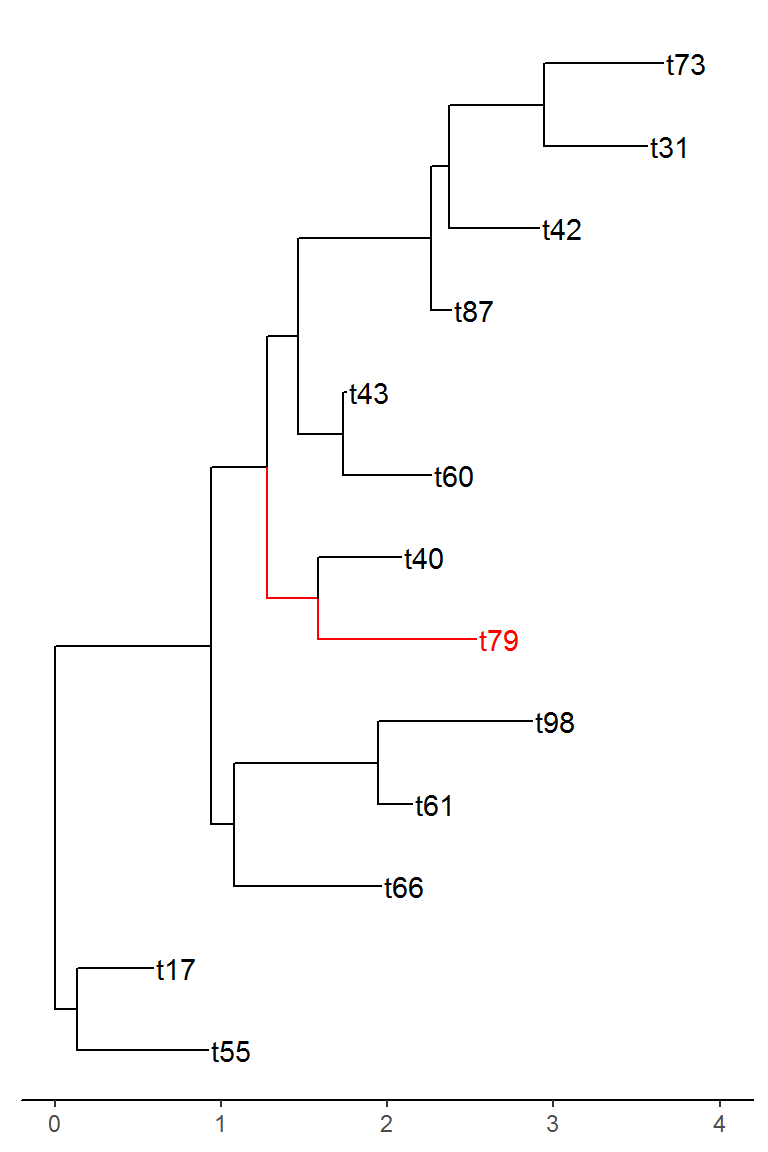Want to share your content on R-bloggers? click here if you have a blog, or here if you don't.

# Introduction

In the past few months, I have been introduced to and started working with phylogenetic trees. The trees I have been working with contain the entire tree of life for bacteria. Needless to say, they are huge. At first, I was told that I could download a program that would let me view the tree and search for particular species on the tree, but of course, I immediately looked for a better solution using R. As is the case for most things, I was able to find a full suite of packages (`treeio`, `ggtree`, and `tidytree` – all created by Guangchuang Yu) that allowed me to interact with this data.

However, since my tree was so large, it was extremely difficult to make legible figures even with the great flexibility of `ggtree`. In order to better analyze the tree as I needed to, I needed to come up with a way to look at only the portions of the tree that I wanted while still maintaining the structure of the tree for the subset portion. As a result, I created the `tree_subset` function which is now in the development version (1.5.1.002) of `treeio` on github. This post will briefly walk through the usage of this function!

Before we get started, let’s load in the required packages.

```# install development version of treeio
# devtools::install_github("GuangchuangYu/treeio")

library(ape)
library(treeio)
library(ggplot2)
library(ggtree)```

# Subset trees by tip label

`tree_subset()` allows the user to subset a tree, either of class `phylo` or `treedata`, by specifying the tip label they are interested in and the number of levels back they want to go. To demonstrate, let’s generate a random tree.

```set.seed(42)

bi_tree <- rtree(100)
bi_tree\$tip_label <- paste0("t", 1:100)```

To show why this function is useful, let’s first try to visualize this tree that has 100 tips.

```bi_tree %>%
ggtree() +
geom_tiplab() +
theme_tree2()```As we can see, the tree can get slightly crowded. Obviously, we could make the figure taller to allow more space for the labels and we could make the text smaller. However, these fixes are not always applicable when you have a lot more than 100 tips. Sometimes you have hundreds or thousands of tips but you are only interested in the portion of the tree around a particular tip. That is where `tree_subset` comes in. Let’s say we are interested in tip `t79` from the above tree and we want to look at the immediate relatives of this tip.

```bi_subset <- tree_subset(bi_tree, "t79", levels_back = 4)

bi_subset %>%
ggtree(aes(color = group)) +
geom_tiplab() +
theme_tree2() +
scale_color_manual(values = c(`1` = "red", `0` = "black")) +
xlim(0, 4)```This function allows for you to easily look at portions of the tree that are of interest. This can greatly reduce the amount of time spent if trying to investigate a large tree for certain species. A couple of important things to note about the function above. As you may have noticed, the specified tip is grouped separately then the rest to allow for it to be easily distinguishable from the rest of the tips. If your tree has existing groups, this functionality can be disabled by specifying `group_node = FALSE` in the `tree_subset` call. Additionally, the branch lengths of the different nodes is maintained after the subset, however, the root of the tree is always anchored at zero for the subset tree, so all distances are relative to this root.

In addition to working on `phylo` objects, this function works also on `treedata` objects and maintains all of the information associated with the `treedata` object. Here is an example with a `treedata` object from the `ggtree` package.

```beast_file <- system.file("examples/MCC_FluA_H3.tree", package="ggtree")
rst_file <- system.file("examples/rst", package="ggtree")
mlc_file <- system.file("examples/mlc", package="ggtree")

merged_tree <- merge_tree(beast_tree, codeml_tree)

merged_tree %>%
ggtree() +
geom_tiplab(size = 3) +
theme_tree2() +
lims(x = c(0, 30))```The tree is pretty crowded when trying to view the full tree. Let’s see what it looks like using `tree_subset`.

```merged_subset <- tree_subset(merged_tree, "A/Swine/GX/2242/2011", levels_back = 4)

merged_subset %>%
ggtree(aes(color = group)) +
geom_tiplab(size = 3) +
scale_color_manual(values = c(`1` = "red", `0` = "black")) +
theme_tree2() +
lims(x = c(0, 4))```# Shiny App to explore phylogentic trees

In addition to the `tree_subset` function in the `treeio` package. I have created a fairly basic shiny application that allows for users to interactively import a tree object and select different nodes that they want to subset the tree by. The code and instructions for running it can be found here. This app allows you to import most of the tree files types that `treeio` has methods for (see here for info on different tree file types). Here is an example of the app:# Conclusion

This function allows users to explore their phylogenetic trees by looking at specific portions of the overall tree when the full tree is too large to easily interpret. The shiny application allows for users who are not familiar with R to easily use this function so that they can explore the trees in a method that, in my opinion, is more efficient than some of the other phylogentic tree programs. I hope that this function is of use to others!

This was my first experience with contributing to an open-source project and Dr. Yu made it extremely easy. I would like to thank him for helping me get my pull request merged and for his great packages!